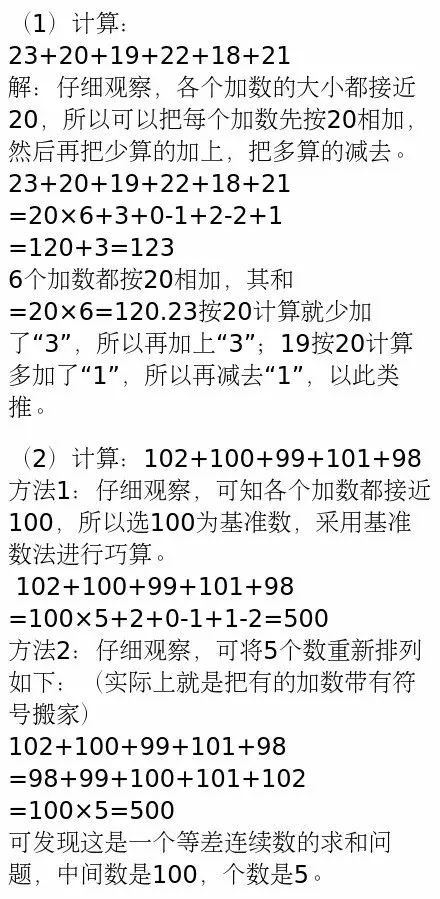>教育>正文

小学数学速算的五种技巧方法，给孩子试试！1

“凑整”先算

1.计算：（1）24+44+56 （2）53+36+47

（2）53+36+47=53+47+36 =（53+47）+36=100+36=136

2.计算：（1）96+15 （2）52+69

=（96+4）+11=100+11=111

（2）52+69=（21+31）+69 =21+（31+69）=21+100=121

3.计算：（1）63+18+19 （2）28+28+28

（2）28+28+28 =（28+2）+（28+2）+（28+2）-6 =30+30+30-6=90-6=84

2

（2）45+18-19=45+（18-19）=45-1=44

3

1，2，3，4，5，6，7，8，9

1，3，5，7，9

2，4，6，8，10

3，6，9，12，15

4，8，12，16，20等等都是等差连续数.

1. 等差连续数的个数是奇数时，它们的和等于中间数乘以个数，简记成：

（1）计算：1+2+3+4+5+6+7+8+9 =5（中间数是5）×9（共9个数）=45

（2）计算：1+3+5+7+9 =5（中间数是5）×5 （共有5个数）=25

（3）计算：2+4+6+8+10 =6（中间数是6 ）×5 （共有5个数）=30

（4）计算：3+6+9+12+15 =9（中间数是9）×5（共有5个数）=45

（5）计算：4+8+12+16+20 =12（中间数是12）×5（共有5个数）=60

2. 等差连续数的个数是偶数时，它们的和等于首数与末数之和乘以个数的一半，简记成：

（1）计算： 1+2+3+4+5+6+7+8+9+10 =（1+10）×5=11×5=55

（2）计算： 3+5+7+9+11+13+15+17 =（3+17）×4=20×4=80

（3）计算： 2+4+6+8+10+12+14+16+18+20 =（2+20）×5=110

4